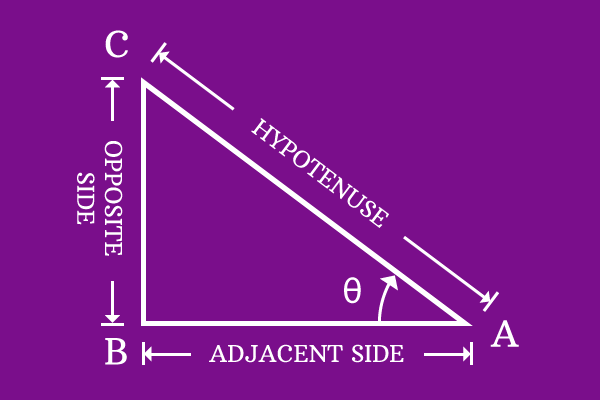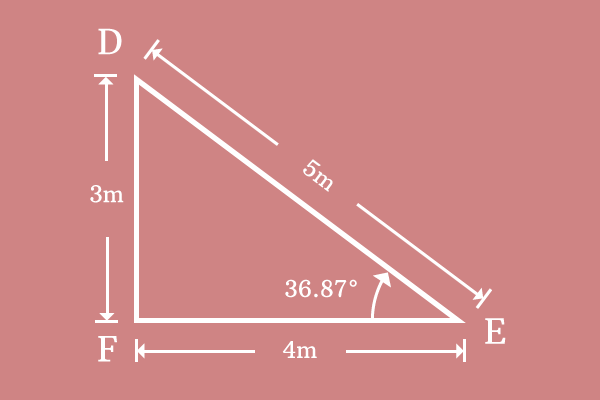# Secant

## Definition

A relation of angle with ratio of length of hypotenuse to length of adjacent side in a right angled triangle is called secant.Secant represents the mutual relation between the angle of right angled triangle and ratio of length of hypotenuse to length of adjacent side of same triangle. In fact, it is defined from the ratio of lengths of two sides, hypotenuse and adjacent side. Hence, secant is also called as trigonometric ratio.

The functionality of representing the relation of angle with ratio of length of hypotenuse to length of adjacent side, is the reason to call secant as trigonometric function in trigonometry.

## Mathematical formIn trigonometry, the name secant was introduced to represent the relation of angle with ratio of length of hypotenuse to length of adjacent side.

The angle and ratio of length of hypotenuse to length of adjacent side are mutually dependable factors because the variation in angle varies the value of ratio of length of hypotenuse to length of adjacent side. Hence, secant is written as secant along with angle but secant is written in its in short form $sec$ in mathematical expressions. The angle of right angled triangle $BAC$ is theta. Therefore, secant along with angle is written as $sec \, \theta$ in mathematical form.

The relation of angle with ratio of length of hypotenuse to length of adjacent side is expressed in mathematical form as follows.

$$sec \, \theta = { Length \, of \, Hypotenuse \over Length \, of \, Adjacent \, Side }$$

In $Δ BAC$, the length of hypotenuse $(\overline{AC})$ is $AC$ and length of adjacent side $(\overline{AB})$ is $AB$ for this triangle. Transform the expression by substituting the lengths of hypotenuse of adjacent side of right angled triangle BAC.

$$sec \, \theta = { AC \over AB }$$

### Example$Δ DEF$ is a right angled triangle. Lengths of hypotenuse, length of adjacent side and angle are measured that

1. The length of hypotenuse $(\overline{DE})$ is $DE = 5$ meters
2. The length of adjacent side $(\overline{EF})$ is $EF = 4$ meters
3. The angle of right angled triangle $DEF$ is $36.87^°$.

$$sec \, 36.87^° = { DE \over EF }$$

Substitute the above three values in the expression of secant.

$$sec \, 36.87^° = { 5 \over 4 }$$

$⇒ \, sec \, 36.87^° = 1.25$

The value of ratio of length of hypotenuse to length of adjacent side is calculated as $1.25$ at the angle $36.87^°$. The value $1.25$ is known as the value of secant at the angle of $36.87^°$. In this way, secant represents the relation between angle and ratio of length of hypotenuse to length of adjacent side.

Latest Math Topics
Latest Math Problems
Email subscription
Math Doubts is a best place to learn mathematics and from basics to advanced scientific level for students, teachers and researchers. Know more